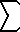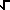Dave's Math Tables: Series Expansions(Math | Calculus | Expansions | Series)

Series (Summation) Expansions

Basic Properties
Convergence Tests
Function-->Summation and Summation-->Function Conversions
 Select function f(x) to expand into a summation  f(x) =? Select term an in summation to simplify:an = ? Exponential / Logarithm Functions    f(x) = e; e-1; e x    f(x) = ln(x)  Root Functions    f(x) =(x); 1/(x) Geometric Series    an = r n  Power Series    an = n; n 2; n 3; ...   an = 1/n; 1/n 2; 1/n 3; 1/n 4; 1/n 5; 1/n 6; 1/n 7; 1/n 8; 1/n 9; 1/n 10; 1/n p